# OpenCV中使用Eigenfaces 或 Fisherfaces进行人脸识别

33 篇文章 0 订阅

OpenCV中使用Eigenfaces 或 Fisherfaces进行人脸识别

Translate by Dawn

~~~~~~~~~~~~~~~~~~~~~~翻译的好辛苦~~~~~~~~~~~~~~~~~~

• 人脸检测

• 人脸预处理培训从收集到的面孔

• 人脸识别

• 收尾介绍

1.人脸检测︰ 是 （在下面的屏幕快照的中心附近的大矩形） 图像中定位人脸区域的过程。这一步不关心谁的人，只是，它是人的脸。

2.脸预处理︰ 它是调整对人脸图像，看起来更清晰，类似于其他的面孔 （在下面的屏幕快照的顶部中心的小灰度脸） 的过程。

3.收集和了解的面孔︰ 这是 （为每一个人应该承认），节省许多预处理过的面孔，然后学习如何识别它们的过程。

4.人脸识别︰ 它是检查哪一种收集人的过程是最类似于在相机 （右上角的下面的截图上的一个小矩形） 的脸。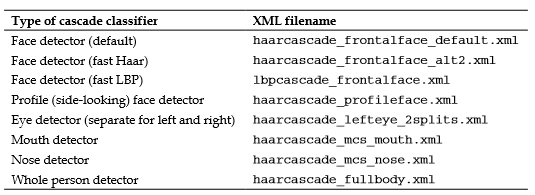try {

} catch (cv::Exception e) {}

if ( faceDetector.empty() ) {

cerr << "ERROR: Couldn't load Face Detector (";

cerr << faceCascadeFilename << ")!" << endl;

exit(1);

}

1.灰度颜色转换︰ 仅适用于灰度图像的人脸检测。所以我们应该将彩色相机帧转换为灰度。

2.摄像机图像收缩︰ 人脸检测的速度取决于输入的图像 （它是非常慢的大图像但快速小图像），大小和尚未检测仍然是相当可靠的即使在低分辨率。所以我们应相机图像缩小到一个更合理的大小 （或使用较大的值在探测器，minFeatureSize，如稍后再作解释）。

3.直方图均衡化︰ 是不可靠在低光照条件下的人脸检测。所以我们应该执行直方图均衡化。

Mat gray;

if (img.channels() == 3) {

cvtColor(img, gray, CV_BGR2GRAY);

} else if (img.channels() == 4) {

cvtColor(img, gray, CV_BGRA2GRAY);

} else {

// Access the grayscale input image directly.

gray = img;

}

const int DETECTION_WIDTH = 320;

// Possibly shrink the image, to run much faster.

Mat smallImg;

loat scale = img.cols / (float) DETECTION_WIDTH;

if (img.cols > DETECTION_WIDTH) {

// Shrink the image while keeping the same aspect ratio.

int scaledHeight = cvRound(img.rows / scale);

resize(img, smallImg, Size(DETECTION_WIDTH, scaledHeight));

} else {

// Access the input directly since it is already small.

smallImg = img;

// Standardize the brightness & contrast, such as

// to improve dark images.

Mat equalizedImg;

equalizeHist(inputImg, equalizedImg);

• minFeatureSize︰ 此参数确定最小脸大小，我们关心的事情，通常 20 × 20 或 30 × 30 像素，但这取决于你使用案例和图像大小。如果您正在执行的人脸检测对摄像头或智能手机，脸上总是将非常接近于相机，你可以放大，这对 80 x 80 有更快的检测，或如果您想要远脸检测，如在海滩上与朋友，然后离开这为 20 × 20。

• searchScaleFactor: 参数确定如何许多不同大小的面孔去寻找;通常它会很好的检测，为 1.1 或 1.2 经常找不到脸的快速检测。

• minNeighbors︰ 此参数确定如何确保探测器应已检测到一张脸，通常值为 3，但您可以设置它高如果你想更可靠的面孔，即使许多面孔不检测。

int flags = CASCADE_SCALE_IMAGE; // Search for many faces.

Size minFeatureSize(20, 20);     // Smallest face

size. float searchScaleFactor = 1.1f;  // How many sizes to search.

int minNeighbors = 4;            // Reliability vs many faces.

// Detect objects in the small grayscale image.

std::vector<Rect> faces;

faceDetector.detectMultiScale(img, faces, searchScaleFactor,

minNeighbors, flags, minFeatureSize);

// Enlarge the results if the image was temporarily shrunk.

if (img.cols > scaledWidth) {

for (int i = 0; i < (int)objects.size(); i++ ) {

objects[i].x = cvRound(objects[i].x * scale);

objects[i].y = cvRound(objects[i].y * scale);

objects[i].width = cvRound(objects[i].width * scale);

objects[i].height = cvRound(objects[i].height * scale);

} // If the object is on a border, keep it in the image.

for (int i = 0; i < (int)objects.size(); i++ ) {

if (objects[i].x < 0)

objects[i].x = 0;

if (objects[i].y < 0)

objects[i].y = 0;

if (objects[i].x + objects[i].width > img.cols)

objects[i].x = img.cols - objects[i].width;

if (objects[i].y + objects[i].height > img.rows)

objects[i].y = img.rows - objects[i].height;

WebcamFaceRec 项目包括 OpenCV 的 Haar 或 LBP 探测器，能容易地找到脸上或眼睛内的图像。例如︰

Rect faceRect;    // Stores the result of the detection, or -1.

int scaledWidth = 320; // Shrink the image before detection.

detectLargestObject(cameraImg, faceDetector, faceRect,

scaledWidth);

if (faceRect.width > 0)

cout << "We detected a face!" << endl;

// Access just the face within the camera image.

Mat faceImg = cameraImg(faceRect);

脸预处理的最简单形式只是将使用 equalizeHist() 函数，像我们只是做了人脸检测的直方图均衡化。这可能是足够的一些项目在哪里的照明和位置的条件不会改变太多。但在现实条件下的可靠性，我们需要很多先进的技术，包括人脸特征检测 （例如，检测眼睛、 鼻子、 嘴和眉毛）。为简单起见，本章将只需使用眼检测，并忽略其他面部的功能，如嘴和鼻子，这是不太有用。下图显示了典型的预处理脸，使用本节中将覆盖技术放大的视图︰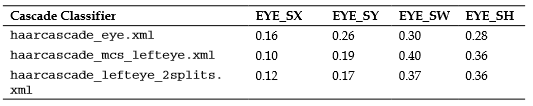int leftX = cvRound(face.cols * EYE_SX);

int topY = cvRound(face.rows * EYE_SY);

int widthX = cvRound(face.cols * EYE_SW);

int heightY = cvRound(face.rows * EYE_SH);

int rightX = cvRound(face.cols * (1.0-EYE_SX-EYE_SW));

Mat topLeftOfFace = faceImg(Rect(leftX, topY, widthX,

heightY));

Mat topRightOfFace = faceImg(Rect(rightX, topY, widthX,

heightY));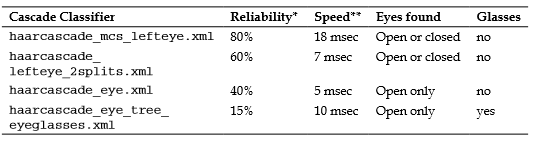* 可靠性值表明多久两只眼睛会检测后 LBP 正面人脸检测时没有眼镜的磨损和两只眼睛都开放。如果闭上眼睛然后可靠性可能会下降，或如果戴眼镜那么可靠性和速度会降低。

* * 速度值是以毫秒为单位的图像缩放到的英特尔酷睿 i7 处理器 320 x 240 像素大小 2.2 GHz （平均值 1000 张照片）。速度通常快很多时如果未发现的眼睛，因为它必须扫描整个图像，但 haarcascade_mcs_lefteye.xml 是仍远低于其他眼探测器比发现的眼睛。

...

Rect leftEyeRect;    // Stores the detected eye.

// Search the left region using the 1st eye detector.

detectLargestObject(topLeftOfFace, eyeDetector1, leftEyeRect,            topLeftOfFace.cols); // If it failed, search the left region using the 2nd eye

// detector.

if (leftEyeRect.width <= 0)

detectLargestObject(topLeftOfFace, eyeDetector2,

leftEyeRect, topLeftOfFace.cols);

// Get the left eye center if one of the eye detectors worked.

Point leftEye = Point(-1,-1);

if (leftEyeRect.width <= 0) {

leftEye.x = leftEyeRect.x + leftEyeRect.width/2 + leftX;

leftEye.y = leftEyeRect.y + leftEyeRect.height/2 + topY;

}

// Do the same for the right-eye ...

// Check if both eyes were detected.

if (leftEye.x >= 0 && rightEye.x >= 0) {

...

}

• 几何变换和裁剪︰ 这一进程包括缩放、 旋转，和翻译图像，眼睛一致的后删除的额头、下巴、 耳朵和从人脸图像的背景。

• 单独直方图均衡化的左、 右两边︰ 这一进程独立标准化的亮度和对比度两边左边和右边的脸。

• 平滑︰ 此过程可以减少使用双边滤波图像噪声。

• 椭圆掩码︰ 椭圆掩码从人脸图像中移除一些剩余的头发和背景。下图显示了预处理步骤 1 到 4 适用于检测人脸的脸。而原来不，请注意最终的图像如何面对，两侧有了好的亮度和对比度: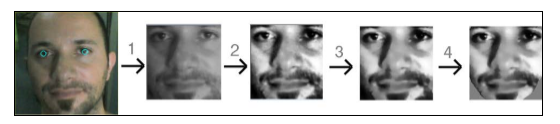• 旋转的脸，两眼的水平。

• 扩展的脸，两只眼睛之间的距离始终不变。

• 翻译的脸，眼睛总是居中水平，所需的高度。

• 作物外部分的脸上，因为我们想要去裁剪图像背景，头发、 额头、 耳朵和下巴。

// Get the center between the 2 eyes.

Point2f eyesCenter;

eyesCenter.x = (leftEye.x + rightEye.x) * 0.5f;

eyesCenter.y = (leftEye.y + rightEye.y) * 0.5f;

// Get the angle between the 2 eyes.

double dy = (rightEye.y - leftEye.y);

double dx = (rightEye.x - leftEye.x);

double len = sqrt(dx*dx + dy*dy);

double angle = atan2(dy, dx) * 180.0/CV_PI;

// Hand measurements shown that the left eye center should

// ideally be roughly at (0.16, 0.14) of a scaled face image.

const double DESIRED_LEFT_EYE_X = 0.16;

const double DESIRED_RIGHT_EYE_X = (1.0f – 0.16);

// Get the amount we need to scale the image to be the desired

// fixed size we want.

const int DESIRED_FACE_WIDTH = 70;

const int DESIRED_FACE_HEIGHT = 70;

double desiredLen = (DESIRED_RIGHT_EYE_X – 0.16);

double scale = desiredLen * DESIRED_FACE_WIDTH / len;

// Get the transformation matrix for the desired angle & size.

Mat rot_mat = getRotationMatrix2D(eyesCenter, angle, scale);

// Shift the center of the eyes to be the desired center.

double ex = DESIRED_FACE_WIDTH * 0.5f - eyesCenter.x;

double ey = DESIRED_FACE_HEIGHT * DESIRED_LEFT_EYE_Y –                      eyesCenter.y;

rot_mat.at<double>(0, 2) += ex;

rot_mat.at<double>(1, 2) += ey;

// Transform the face image to the desired angle & size &

// position! Also clear the transformed image background to a

// default grey.

Mat warped = Mat(DESIRED_FACE_HEIGHT, DESIRED_FACE_WIDTH,                     CV_8U, Scalar(128));

warpAffine(gray, warped, rot_mat, warped.size());

int w = faceImg.cols; int h = faceImg.rows; Mat wholeFace; equalizeHist(faceImg,  wholeFace);

int midX = w/2;

Mat leftSide = faceImg(Rect(0,0, midX,h));

Mat rightSide = faceImg(Rect(midX,0, w-midX,h));

equalizeHist(leftSide, leftSide);

equalizeHist(rightSide, rightSide);

for (int y=0; y<h; y++) {

for (int x=0; x<w; x++) {

int v;

if (x < w/4) {

// Left 25%: just use the left face.

v = leftSide.at<uchar>(y,x);

}  else if (x < w*2/4) {

// Mid-left 25%: blend the left face & whole face.

int lv = leftSide.at<uchar>(y,x);

int wv = wholeFace.at<uchar>(y,x);

// Blend more of the whole face as it moves

// further right along the face.

float f = (x - w*1/4) / (float)(w/4);

v = cvRound((1.0f - f) * lv + (f) * wv);

}  else if (x < w*3/4) {

// Mid-right 25%: blend right face & whole face.

int rv = rightSide.at<uchar>(y,x-midX);

int wv = wholeFace.at<uchar>(y,x);

// Blend more of the right-side face as it moves

// further right along the face.

float f = (x - w*2/4) / (float)(w/4);

v = cvRound((1.0f - f) * wv + (f) * rv);

}  else {

// Right 25%: just use the right face.

v = rightSide.at<uchar>(y,x-midX);

}

faceImg.at<uchar>(y,x) = v;

}// end x loop

}//end y loop

Mat filtered = Mat(warped.size(), CV_8U); bilateralFilter(warped, filtered, 0, 20.0, 2.0);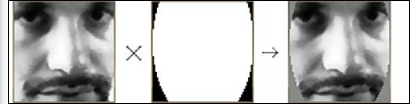// Draw a black-filled ellipse in the middle of the image.

// First we initialize the mask image to white (255).

Mat mask = Mat(warped.size(), CV_8UC1, Scalar(255));

double dw = DESIRED_FACE_WIDTH;

double dh = DESIRED_FACE_HEIGHT;

Point faceCenter = Point( cvRound(dw * 0.5),

cvRound(dh * 0.4) );

Size size = Size( cvRound(dw * 0.5), cvRound(dh * 0.8) );

ellipse(mask, faceCenter, size, 0, 0, 360, Scalar(0),

CV_FILLED);

// Apply the elliptical mask on the face, to remove corners.

// Sets corners to gray, without touching the inner face.

// Check how long since the previous face was added.

double current_time = (double)getTickCount();

double timeDiff_seconds = (current_time –

old_time) / getTickFrequency();

double getSimilarity(const Mat A, const Mat B) {

// Calculate the L2 relative error between the 2 images.

double errorL2 = norm(A, B, CV_L2);

// Scale the value since L2 is summed across all pixels.

double similarity = errorL2 / (double)(A.rows * A.cols);

return similarity;

}

...

// Check if this face looks different from the previous face.

double imageDiff = MAX_DBL;

if (old_prepreprocessedFaceprepreprocessedFace.data) {

ImageDiff = getSimilarity(preprocessedFace,                           old_prepreprocessedFace);

}

// Only process the face if it's noticeably different from the

// previous frame and there has been a noticeable time gap.

if ((imageDiff > 0.3) && (timeDiff_seconds > 1.0)) {

// Also add the mirror image to the training set.

Mat mirroredFace;

flip(preprocessedFace, mirroredFace, 1);

// Add the face & mirrored face to the detected face lists.    preprocessedFaces.push_back(preprocessedFace);     preprocessedFaces.push_back(mirroredFace);

faceLabels.push_back(m_selectedPerson);

faceLabels.push_back(m_selectedPerson);

// Keep a copy of the processed face,

// to compare on next iteration.

old_prepreprocessedFace = preprocessedFace;

old_time = current_time;

}

// Get access to the face region-of-interest.

Mat displayedFaceRegion = displayedFrame(faceRect);

// Add some brightness to each pixel of the face region.

displayedFaceRegion += CV_RGB(90,90,90);

• Eigenfaces  (也被称为主成分分析 (PCA)

• Fisherfaces (也被称为线性判别分析 (LDA)

• 其他经典的人脸识别算法（许多是发售在

http://www.face-rec.org/algorithms/）

• Newer face对最近的计算机视觉研究论文（如视觉和 ICCV 在 http://www.cvpapers.com/） 识别算法那里数以百计的脸识别论文每年出版然而，你不需要去了解这些算法的理论内容，使用它们，在这本书中所示。由于 OpenCV 团队和菲利普·瓦格纳 libfacerec 贡献，OpenCV v2.4.1 提供 cv::Algorithm 作为一种简单通用的方法来执行使用几种不同的算法（在运行时甚至可选） 之一不一定理解它们如何付诸实施的人脸识别。通过算法︰ getList() 功能，如使用此代码，可以在 OpenCV 你版本中找到可用算法︰

vector<string> algorithms;

Algorithm::getList(algorithms);

cout << "Algorithms: " << algorithms.size() << endl;

for (int i=0; i<algorithms.size(); i++) {

cout << algorithms[i] << endl;

}

• FaceRecognizer.Eigenfaces︰  Eigenfaces，也被称为 PCA，特克和彭特兰在 1991 年第一次使用。

• FaceRecognizer.Fisherfaces: Fisherfaces，也被称为 LDA，1997 年发明的 Belhumeur、 Hespanha 和 Kriegman。

• FaceRecognizer.LBPH︰ 本地二进制模式直方图，2004 年由阿、 哈迪德和 Pietikäinen 发明。

// Load the "contrib" module is dynamically at runtime.

bool haveContribModule = initModule_contrib();

if (!haveContribModule) {

cerr << "ERROR: The 'contrib' module is needed for ";

cerr << "FaceRecognizer but hasn't been loaded to OpenCV!";

cerr << endl;    exit(1);

string facerecAlgorithm = "FaceRecognizer.Fisherfaces";

Ptr<FaceRecognizer> model;

// Use OpenCV's new FaceRecognizer in the "contrib" module:

model = Algorithm::create<FaceRecognizer>(facerecAlgorithm);

if (model.empty()) {

cerr << "ERROR: The FaceRecognizer [" << facerecAlgorithm;

cerr << "] is not available in your version of OpenCV. ";

exit(1);

}

// Do the actual training from the collected faces. model->train(preprocessedFaces, faceLabels);

// Convert the matrix row or column (float matrix) to a

// rectangular 8-bit image that can be displayed or saved.

// Scales the values to be between 0 to 255.

Mat getImageFrom1DFloatMat(const Mat matrixRow, int height) {

// Make a rectangular shaped image instead of a single row.

Mat rectangularMat = matrixRow.reshape(1, height);

// Scale the values to be between 0 to 255 and store them

// as a regular 8-bit uchar image.

Mat dst;

normalize(rectangularMat, dst, 0, 255, NORM_MINMAX,                      CV_8UC1);

return dst;

Mat img = ...; printMatInfo(img, "My Image");

My Image: 640w480h 3ch 8bpp, range[79,253][20,58][18,87]

Average face

Mat averageFace = model->get<Mat>("mean");

printMatInfo(averageFace, "averageFace (row)");

// Convert a 1D float row matrix to a regular 8-bit image.

averageFace = getImageFrom1DFloatMat(averageFace, faceHeight);  printMatInfo(averageFace, "averageFace");

imshow("averageFace", averageFace);

averageFace (row): 4900w1h 1ch 64bpp, range[5.21,251.47]

averageFace: 70w70h 1ch 8bpp, range[0,255]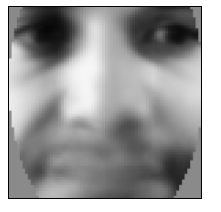Mat eigenvalues = model->get<Mat>("eigenvalues");

printMat(eigenvalues, "eigenvalues");

eigenvalues: 1w18h 1ch 64bpp, range[4.52e+04,2.02836e+06]

2.03e+06

1.09e+06

5.23e+05

4.04e+05

2.66e+05

2.31e+05

1.85e+05

1.23e+05

9.18e+04

7.61e+04

6.91e+04

4.52e+04

eigenvalues: 2w1h 1ch 64bpp, range[152.4,316.6]

317, 152

// Get the eigenvectors

Mat eigenvectors = model->get<Mat>("eigenvectors"); printMatInfo(eigenvectors, "eigenvectors");

// Show the best 20 eigenfaces

for (int i = 0; i < min(20, eigenvectors.cols); i++) {

// Create a continuous column vector from eigenvector #i.

Mat eigenvector = eigenvectors.col(i).clone();

Mat eigenface = getImageFrom1DFloatMat(eigenvector,                                faceHeight);

imshow(format("Eigenface%d", i), eigenface);

}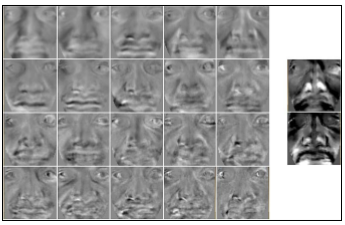int identity = model->predict(preprocessedFace);

OpenCV 的 FaceRecognizer 类可以返回信心指标。我们将使用的方法是使用特征向量和特征值，面部图像重建和比较这重建的图像与输入图像。如果人有很多他们列入训练集的脸，然后重建应该工作得很好从学特征向量和特征值，但是如果人没有任何的面孔在训练集 （或没有任何包含类似照明和面部表情作为测试图像），然后重建的脸会看非常不同于输入的脸信号，它可能不熟悉的面孔。 还记得我们刚才所说的特征脸和 Fisherfaces 算法基于图像可以大致为代表，作为一套 （特别人脸图像） 的特征向量和特征值 （混合比率） 的概念。所以假如我们结合的训练集的面孔之一特征值的特征向量，我们就应获得相当密切那原始的训练图像的副本。这同样适用与其他类似的训练集的图像 — — 如果我们结合从类似的测试图像特征值的受过训练的特征向量，我们应该能够重建图像的某种程度上对测试图像副本。

// Get some required data from the FaceRecognizer model.

Mat eigenvectors = model->get<Mat>("eigenvectors");

Mat averageFaceRow = model->get<Mat>("mean");

// Project the input image onto the eigenspace.

Mat projection = subspaceProject(eigenvectors, averageFaceRow,                    preprocessedFace.reshape(1,1));

// Generate the reconstructed face back from the eigenspace.

Mat reconstructionRow = subspaceReconstruct(eigenvectors,                    averageFaceRow, projection);

// Make it a rectangular shaped image instead of a single row.

Mat reconstructionMat = reconstructionRow.reshape(1,

faceHeight);

// Convert the floating-point pixels to regular 8-bit uchar. Mat reconstructedFace = Mat(reconstructionMat.size(), CV_8U);

reconstructionMat.convertTo(reconstructedFace, CV_8U, 1, 0);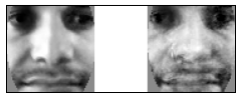similarity = getSimilarity(preprocessedFace,                           reconstructedFace);

if (similarity > UNKNOWN_PERSON_THRESHOLD) {

identity = -1;

// Unknown person.

}

model->save("trainedModel.yml");

string facerecAlgorithm = "FaceRecognizer.Fisherfaces";

model = Algorithm::create<FaceRecognizer>(facerecAlgorithm);

Mat labels;

try {

labels = model->get<Mat>("labels");

} catch (cv::Exception &e) {}

if (labels.rows <= 0) {

cerr << "ERROR: Couldn't load trained data from "

"[trainedModel.yml]!" << endl;

exit(1);

• 启动︰ 这种状态加载并初始化数据和网络摄像头。

• 检测︰ 这种状态检测的面孔和显示他们与预处理，直到用户单击添加人员按钮。

• 收集︰ 这种状态收集面临当前的人，直到用户在窗口中单击任何位置。这也表明每个人的最新面貌。用户单击某一现有人或添加人员按钮，为不同的人收集的面孔。

• 培训︰ 在此状态下，所有收集的脸，所有收集到的人的帮助训练系统。

• 识别︰ 这包括突出的公认的人和显示信心米。在用户单击的人之一或添加人员按钮，以返回到模式 2 （集合）。

// Draw text into an image. Defaults to top-left-justified

// text, so give negative x coords for right-justified text,

// and/or negative y coords for bottom-justified text.

// Returns the bounding rect around the drawn text.

Rect drawString(Mat img, string text, Point coord, Scalar

color, float fontScale = 0.6f, int thickness = 1,

int fontFace = FONT_HERSHEY_COMPLEX);

// Draw it as black shadow & again as white text. float txtSize = 0.4;

int BORDER = 10;

drawString(displayedFrame, msg, Point(BORDER, -BORDER-2),             CV_RGB(0,0,0), txtSize);

Rect rcHelp = drawString(displayedFrame, msg, Point(BORDER+1,             -BORDER-1), CV_RGB(255,255,255), txtSize);

// Draw a GUI button into the image, using drawString().

// Can give a minWidth to have several buttons of same width.

// Returns the bounding rect around the drawn button.

Rect drawButton(Mat img, string text, Point coord,

int minWidth = 0)

{

const int B = 10;

Point textCoord = Point(coord.x + B, coord.y + B);

// Get the bounding box around the text.

Rect rcText = drawString(img, text, textCoord,                     CV_RGB(0,0,0));

// Draw a filled rectangle around the text.

Rect rcButton = Rect(rcText.x - B, rcText.y – B,

rcText.width + 2*B, rcText.height + 2*B);

// Set a minimum button width.

if (rcButton.width < minWidth)

rcButton.width = minWidth;

// Make a semi-transparent white rectangle.

Mat matButton = img(rcButton);

matButton += CV_RGB(90, 90, 90);

// Draw a non-transparent white border.

rectangle(img, rcButton, CV_RGB(200,200,200), 1, CV_AA);

// Draw the actual text that will be displayed.

drawString(img, text, textCoord, CV_RGB(10,55,20));

return rcButton;

}

// Create a GUI window for display on the screen.

namedWindow(windowName);

// Call "onMouse()" when the user clicks in the window.

setMouseCallback(windowName, onMouse, 0);

// Set the camera resolution. Only works for some systems.  videoCapture.set(CV_CAP_PROP_FRAME_WIDTH, 640); videoCapture.set(CV_CAP_PROP_FRAME_HEIGHT, 480);

// We're already initialized, so let's start in Detection mode.

m_mode = MODE_DETECTION;

• Mat preprocessedFace︰ 预处理的脸 （如果检测到的脸和眼睛）

• Rect faceRect︰ 检测到人脸区域坐标

• 点拍摄，rightEye︰ 检测到左、 右眼中心的坐标

bool gotFaceAndEyes = false;

if (preprocessedFace.data)

gotFaceAndEyes = true;

if (faceRect.width > 0) {

// Draw an anti-aliased rectangle around the detected face.    rectangle(displayedFrame, faceRect, CV_RGB(255, 255, 0), 2,                CV_AA);

// Draw light-blue anti-aliased circles for the 2 eyes.

Scalar eyeColor = CV_RGB(0,255,255);

if (leftEye.x >= 0) {

// Check if the eye was detected

circle(displayedFrame, Point(faceRect.x + leftEye.x,                    faceRect.y + leftEye.y), 6, eyeColor, 1, CV_AA);

} if (rightEye.x >= 0) {

// Check if the eye was detected

circle(displayedFrame, Point(faceRect.x + rightEye.x,                    faceRect.y + rightEye.y), 6, eyeColor, 1, CV_AA);

}

int cx = (displayedFrame.cols - faceWidth) / 2;

if (preprocessedFace.data) {

// Get a BGR version of the face, since the output is BGR.

Mat srcBGR = Mat(preprocessedFace.size(), CV_8UC3);

cvtColor(preprocessedFace, srcBGR, CV_GRAY2BGR);

// Get the destination ROI.

Rect dstRC = Rect(cx, BORDER, faceWidth, faceHeight);

Mat dstROI = displayedFrame(dstRC);

// Copy the pixels from src to dst.

srcBGR.copyTo(dstROI);

// Draw an anti-aliased border around the face.

rectangle(displayedFrame, Rect(cx-1, BORDER-1, faceWidth+2,               faceHeight+2), CV_RGB(200,200,200), 1, CV_AA);

// Keep a reference to the latest face of each person.

m_latestFaces[m_selectedPerson] = preprocessedFaces.size() - 2;

m_gui_faces_left = displayedFrame.cols - BORDER - faceWidth;

m_gui_faces_top = BORDER; for (int i=0; i<m_numPersons; i++) {

int index = m_latestFaces[i];

if (index >= 0 && index < (int)preprocessedFaces.size()) {

Mat srcGray = preprocessedFaces[index];

if (srcGray.data) {

// Get a BGR face, since the output is BGR.

Mat srcBGR = Mat(srcGray.size(), CV_8UC3);

cvtColor(srcGray, srcBGR, CV_GRAY2BGR);

// Get the destination ROI

int y = min(m_gui_faces_top + i * faceHeight,                        displayedFrame.rows - faceHeight);

Rect dstRC = Rect(m_gui_faces_left,  y,  faceWidth,  faceHeight);

Mat dstROI = displayedFrame(dstRC);

// Copy the pixels from src to dst.

srcBGR.copyTo(dstROI);

}

}

if (m_mode == MODE_COLLECT_FACES) {

if (m_selectedPerson >= 0 && m_selectedPerson < m_numPersons) {

int y = min(m_gui_faces_top + m_selectedPerson * faceHeight,

displayedFrame.rows – faceHeight);

Rect rc = Rect(m_gui_faces_left,  y,  faceWidth,  faceHeight);        rectangle(displayedFrame, rc, CV_RGB(255, 0, 0),  3, CV_AA);

}

// Check if there is enough data to train from.

bool haveEnoughData = true;

if (!strcmp(facerecAlgorithm, "FaceRecognizer.Fisherfaces")) {

if ((m_numPersons < 2) || (m_numPersons == 2 && m_latestFaces < 0) ) {

cout << "Fisherfaces needs >= 2 people!" << endl;

haveEnoughData = false;

} if (m_numPersons < 1 || preprocessedFaces.size() <= 0 ||            preprocessedFaces.size() != faceLabels.size()) {

cout << "Need data before it can be learnt!" << endl;    haveEnoughData = false; }

if (haveEnoughData) {

// Train collected faces using Eigenfaces or Fisherfaces.

model = learnCollectedFaces(preprocessedFaces, faceLabels,                 facerecAlgorithm);

// Now that training is over, we can start recognizing!

m_mode = MODE_RECOGNITION;

} else {

// Not enough training data, go back to Collection mode!

m_mode = MODE_COLLECT_FACES;

int cx = (displayedFrame.cols - faceWidth) / 2;

Point ptBottomRight = Point(cx - 5, BORDER + faceHeight);

Point ptTopLeft = Point(cx - 15, BORDER);

// Draw a gray line showing the threshold for "unknown" people.

Point ptThreshold = Point(ptTopLeft.x, ptBottomRight.y(1.0 -  UNKNOWN_PERSON_THRESHOLD) * faceHeight);

rectangle(displayedFrame, ptThreshold, Point(ptBottomRight.x,                ptThreshold.y), CV_RGB(200,200,200), 1, CV_AA);

// Crop the confidence rating between 0 to 1 to fit in the bar.

double confidenceRatio = 1.0 - min(max(similarity, 0.0), 1.0);

Point ptConfidence = Point(ptTopLeft.x, ptBottomRight.y                  confidenceRatio * faceHeight);

// Show the light-blue confidence bar.

rectangle(displayedFrame, ptConfidence, ptBottomRight,                CV_RGB(0,255,255), CV_FILLED, CV_AA);

// Show the gray border of the bar.

rectangle(displayedFrame, ptTopLeft, ptBottomRight,                   CV_RGB(200,200,200), 1, CV_AA);

if (identity >= 0 && identity < 1000) {

int y = min(m_gui_faces_top + identity * faceHeight,                    displayedFrame.rows - faceHeight);

Rect rc = Rect(m_gui_faces_left, y, faceWidth, faceHeight);

rectangle(displayedFrame, rc, CV_RGB(0,255,0), 3, CV_AA);

}

void onMouse(int event, int x, int y, int, void*) {

if (event != CV_EVENT_LBUTTONDOWN)

return;

Point pt = Point(x,y);

... (handle mouse clicks) ...

// Ensure there isn't a person without collected faces.

if ((m_numPersons==0) || (m_latestFaces[m_numPersons-1] >= 0)) {

m_numPersons++;

m_latestFaces.push_back(-1);

}

m_selectedPerson = m_numPersons - 1;

m_mode = MODE_COLLECT_FACES;

else if (pt.inside(m_btnDebug)) {

m_debug = !m_debug;

}

// Ensure there isn't a person without collected faces.

if ((m_numPersons==0) || (m_latestFaces[m_numPersons-1] >= 0)) {

m_numPersons++;

m_latestFaces.push_back(-1);

}

m_selectedPerson = m_numPersons - 1;

m_mode = MODE_COLLECT_FACES;

else if (pt.inside(m_btnDebug)) {

m_debug = !m_debug;

}

else {

// Check if the user clicked on a face from the list.

int clickedPerson = -1;

for (int i=0; i<m_numPersons; i++) {

if (m_gui_faces_top >= 0) {

Rect rcFace = Rect(m_gui_faces_left,

m_gui_faces_top + i * faceHeight, faceWidth, faceHeight);

if (pt.inside(rcFace)) {

clickedPerson = i;

break;

}

}

}

// Change the selected person, if the user clicked a face.

if (clickedPerson >= 0) {

// Change the current person & collect more photos.

m_selectedPerson = clickedPerson;

m_mode = MODE_COLLECT_FACES;

}

// Otherwise they clicked in the center.

else {

// Change to training mode if it was collecting faces.

if (m_mode == MODE_COLLECT_FACES) {

m_mode = MODE_TRAINING;

}

}

• Rapid Object Detection using a Boosted Cascade of Simple Features, P. Viola  and M.J. Jones, Proceedings of the IEEE Transactions on CVPR 2001, Vol. 1,  pp. 511-518

• An Extended Set of Haar-like Features for Rapid Object Detection, R. Lienhart and J. Maydt, Proceedings of the IEEE Transactions on ICIP 2002, Vol. 1, pp. 900-903

• Face Description with Local Binary Patterns: Application to Face Recognition, T. Ahonen, A. Hadid and M. Pietikäinen, Proceedings of the IEEE Transactions on PAMI 2006, Vol. 28, Issue 12, pp. 2037-2041

• Learning OpenCV: Computer Vision with the OpenCV Library, G. Bradski and A. Kaehler, pp. 186-190, O'Reilly Media.

• Eigenfaces for recognition, M. Turk and A. Pentland, Journal of Cognitive Neuroscience 3, pp. 71-86

• Eigenfaces vs. Fisherfaces: Recognition using class specific linear projection, P.N. Belhumeur, J. Hespanha and D. Kriegman, Proceedings of the IEEE Transactions on PAMI 1997, Vol. 19, Issue 7, pp. 711–720

• Face Recognition with Local Binary Patterns, T. Ahonen, A. Hadid and M. Pietikäinen, Computer Vision - ECCV 2004, pp. 469–48

09-29270509-13799
06-091197
02-275707
01-22922
07-302万+
07-081425
07-292万+
04-095061
04-108609
11-126397
08-037万+
07-187355
08-07486
05-145万+
05-288531点击重新获取扫码支付余额充值